TitleBeginning Algebra
Tutorial 10: Practice Test on Tutorials 1 - 9Learning Objectives

 After completing this tutorial, you should be able to: Take a test on topics covered in tutorials 1 - 9 in this website.

 Special Notes about Tutorial 10: I can not guarantee you will pass your test after going though any of the tutorials in this website or this practice test. However, it will definitely help you to better understand the topics covered better. Disclaimer: WTAMU and Kim Seward are not responsible for how a student does on any test or any class for any reason including not being able to access the website due to any technology problems.  There are no videos on this page.Introduction

It is important to note that, chances are, I'm not your math instructor. You need to check with your math teacher as to things like when your next math test is and what it covers.  It may cover more material on the test than what is in this practice test.  Just note that there are other practice tests at this website. So, after finding out what is on your test (if you have one) do the practice test(s) problems that go with the test you are preparing for.  If you are not in a class or are not having a test soon, this practice test is still good practice to go through and check to make sure you are understanding this material before moving on - kind of like a spot check. The material on this practice test goes with Tutorial 2:  Symbols and Sets of Numbers, Tutorial 3:  Fractions, Tutorial 4:  Introduction to Variable Expressions and Equations, Tutorial 5: Adding Real Numbers, Tutorial 6: Subtracting Real Numbers, Tutorial 7: Multiplying and Dividing Real Numbers, Tutorial 8: Properties of Real Numbers, Tutorial 9: Reading Graphs.

Also note that your teacher may word the problems on their test a little differently, may have some different kinds of problems, or may have a different number of problems than what is in this practice test.  Again, since I'm probably not your math instructor, I don't know exactly how your teacher will set up your math test.  Just note that these problems will help you build an understanding of the concepts presented and the terms used in math problems.  If you have an understanding of the problems instead of just memorizing them, then you should do fine on these concepts, no matter how the test is set up.

 Steps to Studying for a Math Test
1. Work through problems.  If you are in a class, you should have done this on completion of any homework you have done.  For anyone,  you can accomplish this by doing the practice problems found in each tutorial.

2. Check work on problems.  The practice problems in each tutorial have links to the answers to them so you can instantly check how you are doing.  Also, in most math books, the odd answers are found in the back of the book.

3. Review concepts.  Whether you got the problems right or wrong, make sure you review over them.  If you did get a problem wrong, make sure you either review that concept in it's respective tutorial or ask your math teacher about it.  If you don't ask about a problem before a test,  you are going to kick yourself when it comes up on the test.

4. Work through problems as if you were taking the test - no notes, book, webpages, etc.  This practice test is a perfect way to do that. After taking this practice test, check your answers by clicking on the link to the answer key found at the bottom of the practice test (before the 'need extra help on these topics' section)

 During the Test

It is to your benefit to show as much of the work as possible on the problems that have several steps involved.

Make sure that you read the directions carefully,  you wouldn't believe how many points get taken off  math tests for people not following directions.

Pace yourself.  You do not have to be the first one done to do well on the test.  Do not panic if there is still time left to take the test and others are turing it in.  Sometimes that means they do not know the material and left some of the answers blank.  Do not worry about anyone else but yourself.

Don't rush through a problem.  Another thing that math teachers take points off for are careless mistakes made by people that rush through a problem.  When those students get their tests back, they bonk themselves on the head at some of the things that got counted wrong,  things that they knew how to do.

Remember to breathe!!!!  I know some of you are scared to death at the thought of having to take a math test of any kind.  For you guys, try to relax and don't forget to breathe.  (Even if you aren't scared to take a math test,  it is probably a good idea to remember to breathe,  I wouldn't want you to pass out during the test). If it feels like your brain has left the building during your test, just close your eyes and breathe in and out and in and out and your brain will return.

Good luck on your test.  If you are taking a math test soon, don't panic, you are going to do great!!!Practice Test

 Problems 1a - 1c: Replace ? with  < , > , or = .

 1a.  -5 ?  5

 1b.  10 ?  5

 1c.  |-5| ? |5|

 Problems 2a - 2b:  Are the following statements true or false?

 2a.  - 4  >  4

 2b.  - 4  <  - 4

 Problems 3a - 3c:  Write each sentence as a mathematical statement.

 3a.  5 is not equal to -5.

 3b.  -2 is less than or equal to 0.

 3c.  7 is greater than 0.

 Problems 4a - 4f :  List the elements of the following set that are also elements of the given set. {-½, 0, 5,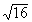,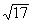}

 4a.  Natural numbers 4b.  Whole numbers

 4c.  Integers 4d.  Rational numbers

 4e.  Irrational numbers 4f.  Real numbers

 Problems 5a:  Write the number as a product of primes.

 5a.  90

 Problems 6a:  Write the fraction in lowest terms.

 6a.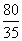Problems 7a - 7d:  Perform the following operations.  Write answers in the lowest terms.

 7a.7b.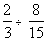7c.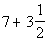7d.Problems 8a - 8b:   Evaluate.

 8a.8b.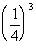Problems 9a - 9b:  Write each phrase as an algebraic expression.  Let x represent the unknown number.

 9a.  The quotient of 7 and a number.

 9b.  10 less than 2 times a number.

 Problems 10a - 10b:  Write each sentence as an equation.  Let x represent the unknown number.

 10a.  The sum of 2 and 10 times a number is the same as 30.

 10b.  The product of 5 and a number is 2/3.

 Problems 11a - 11c:  Add.

 11a.  -9 + (-10) 11b.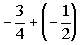11c.  6.5 + (-1.2) + (-3.1)

 Problems 12a - 12b: Simplify.

 12a.  -(-17) 12b.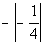Problems 13a - 13b: Subtract.

 13a.  -15 - (-3) 13b.  -1.5 - 2.5

 Problems 14a - 14c:  Multiply.

 14a.  (-5)(-12) 14b.  (3)(-5)(2)

 14c.  (-15)(0)

 Problems 15a - 15c: Divide.

 15a.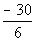15b.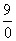15c.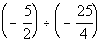Problems 16a - 16b:   Simplify.

 16a.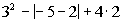16b.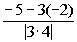Problems 17a - 17b:   Evaluate the expression.

 17a.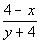when x = 3 and y = -3.

 17b.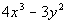when x = 2 and y = -2

 Problems 18a - 18b: Decide whether the given number is a solution of the given equation.

 18a.  Is 1 a solution to 3x - 1 = 4?

 18b.  Is -3 a solution to 7 - x = 2x + 16?

 Problem 19a:  Use a commutative property to write an  equivalent expression.

 19a.  3a + 2b

 Problem 20a:  Use an associative property to write an  equivalent expression.

 20a.  8(xy)

 Problems 21a - 21b:  Use the distributive property to find the product.

 21a.    -5(a - 7) 21b.  8(2x + 3y +4z)

 Problems 22a - 22b: Write the opposite (additive inverse) and the reciprocal (multiplicative inverse) of each number.

 22a.  -10 22b.Problem 23a - 23c:   The bar graph below shows the profit a cd store made over the months of September through December of last year.   Use the graph to answer questions 23a - 23c.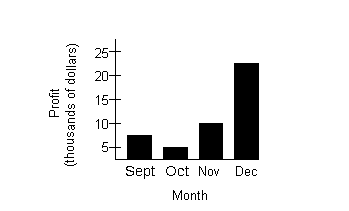23a.  About how much was the profit in December?  23b.  Which month had the lowest profit? 23c.  What is the sum of the profits of October and November?

 Problems 24a - 24b: The line graph below shows last week's high temperatures in Fahrenheit.   Use the graph to answer questions 24a - 24b.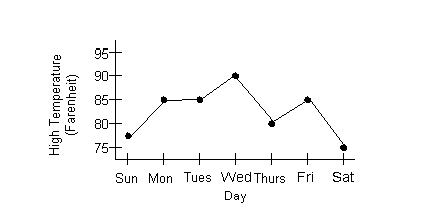24a.  How much was Monday's high temperature?  24b.  Which day had the highest high temperature?

 Now you are ready to check the answers to your practice test:Need Extra Help on these Topics?

The following are web pages that can assist you in the topics that were covered on this page:

Go to Get Help Outside the Classroom found in Tutorial 1: How to Succeed in a Math Class for some more suggestions.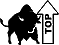Last revised on July 25, 2011 by Kim Seward.
All contents copyright (C) 2002 - 2010, WTAMU and Kim Seward. All rights reserved.